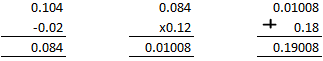# Selina Solutions Concise Maths Class 7 Chapter 4: Decimal Fractions (Decimals) Exercise 4F

Selina Solutions Concise Maths Class 7 Chapter 4 Decimal Fractions (Decimals) Exercise 4F are prepared by teachers at BYJU’S having experience in the education industry. The main aim of providing solutions is to help students, irrespective of their intelligence quotient. The solutions have explanations in simple language to help students perform well in the annual exam. Students are advised to solve the exercise wise problems on a daily basis to understand the concepts in a better way. Students can download Selina Solutions Concise Maths Class 7 Chapter 4 Decimal Fractions (Decimals) Exercise 4F PDF from the links, which are given here.

## Selina Solutions Concise Maths Class 7 Chapter 4: Decimal Fractions (Decimals) Exercise 4F Download PDF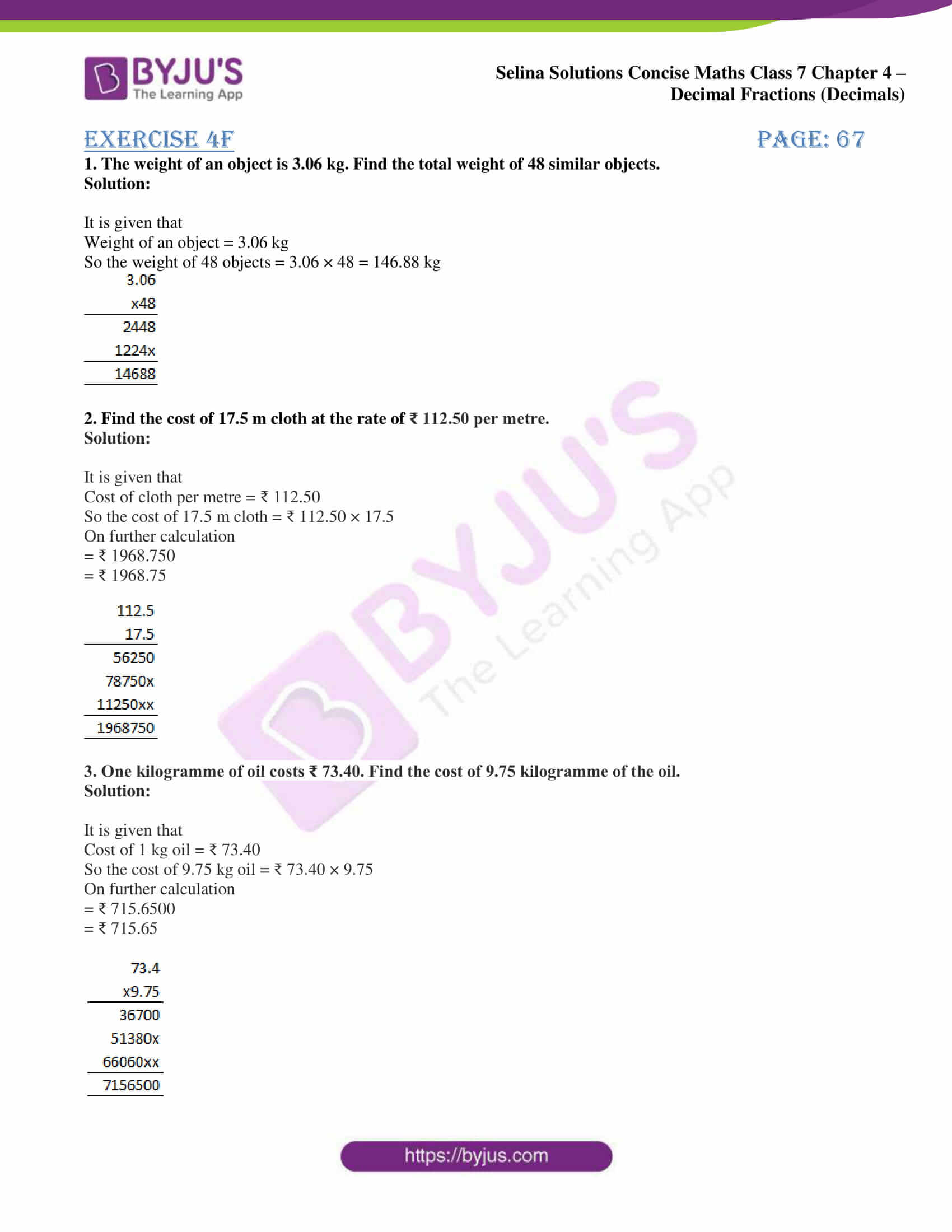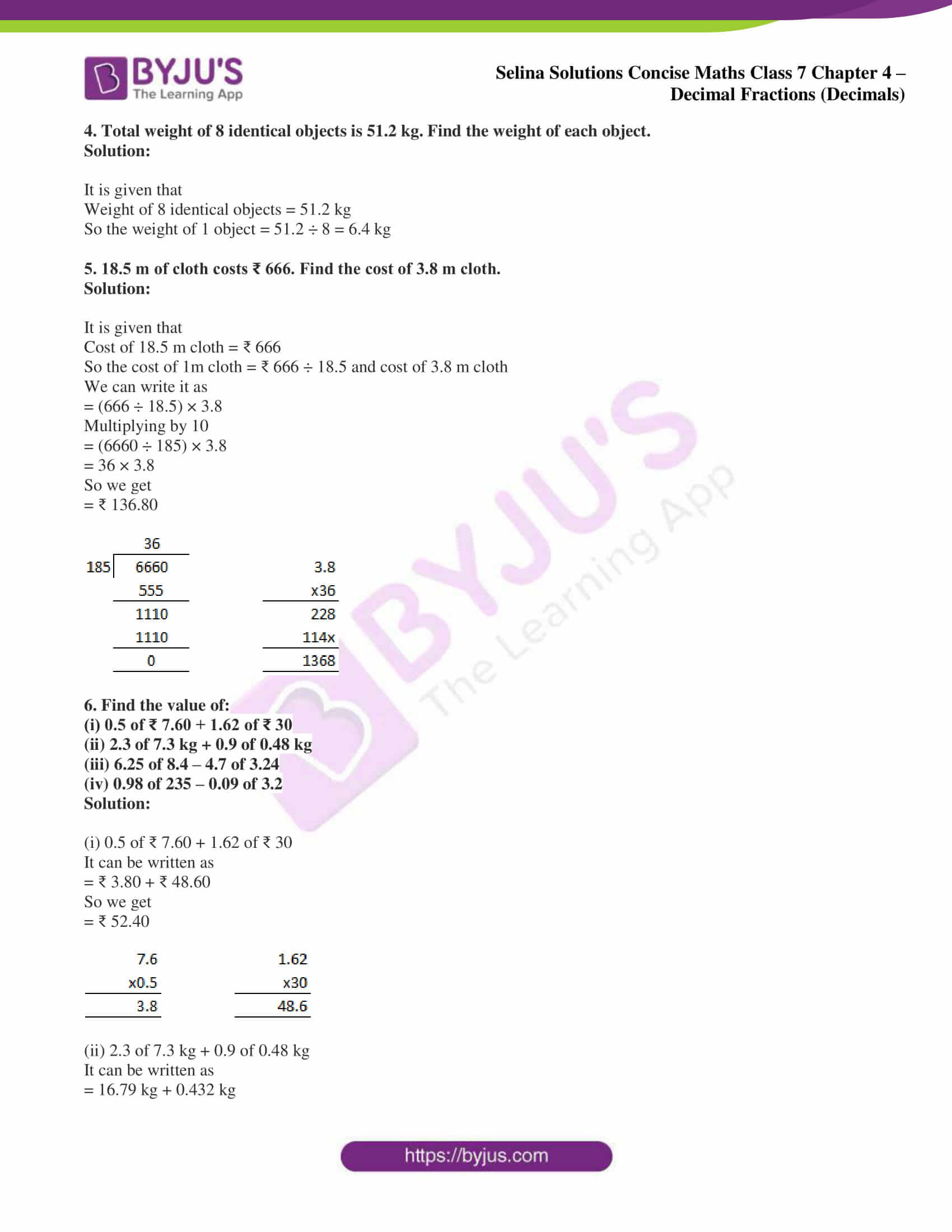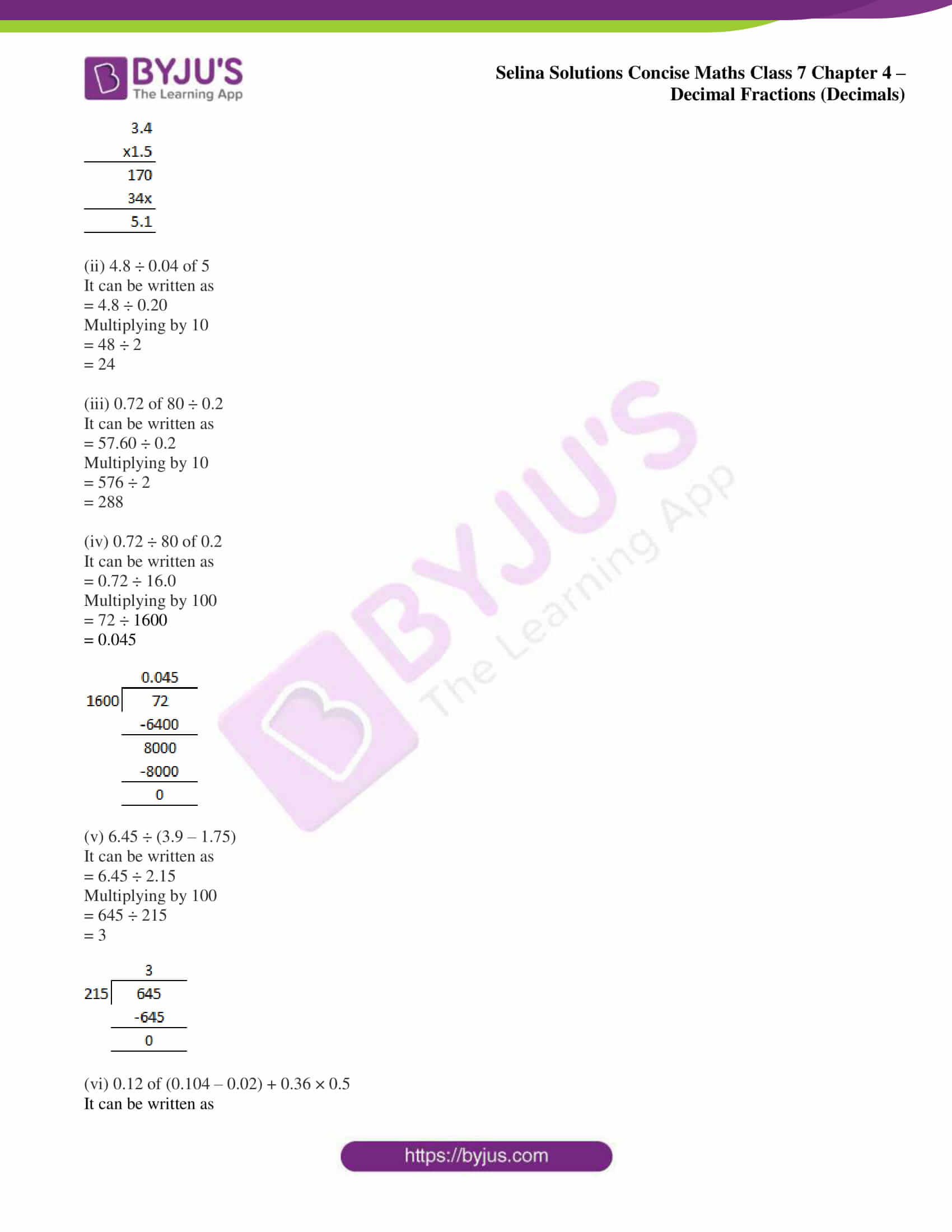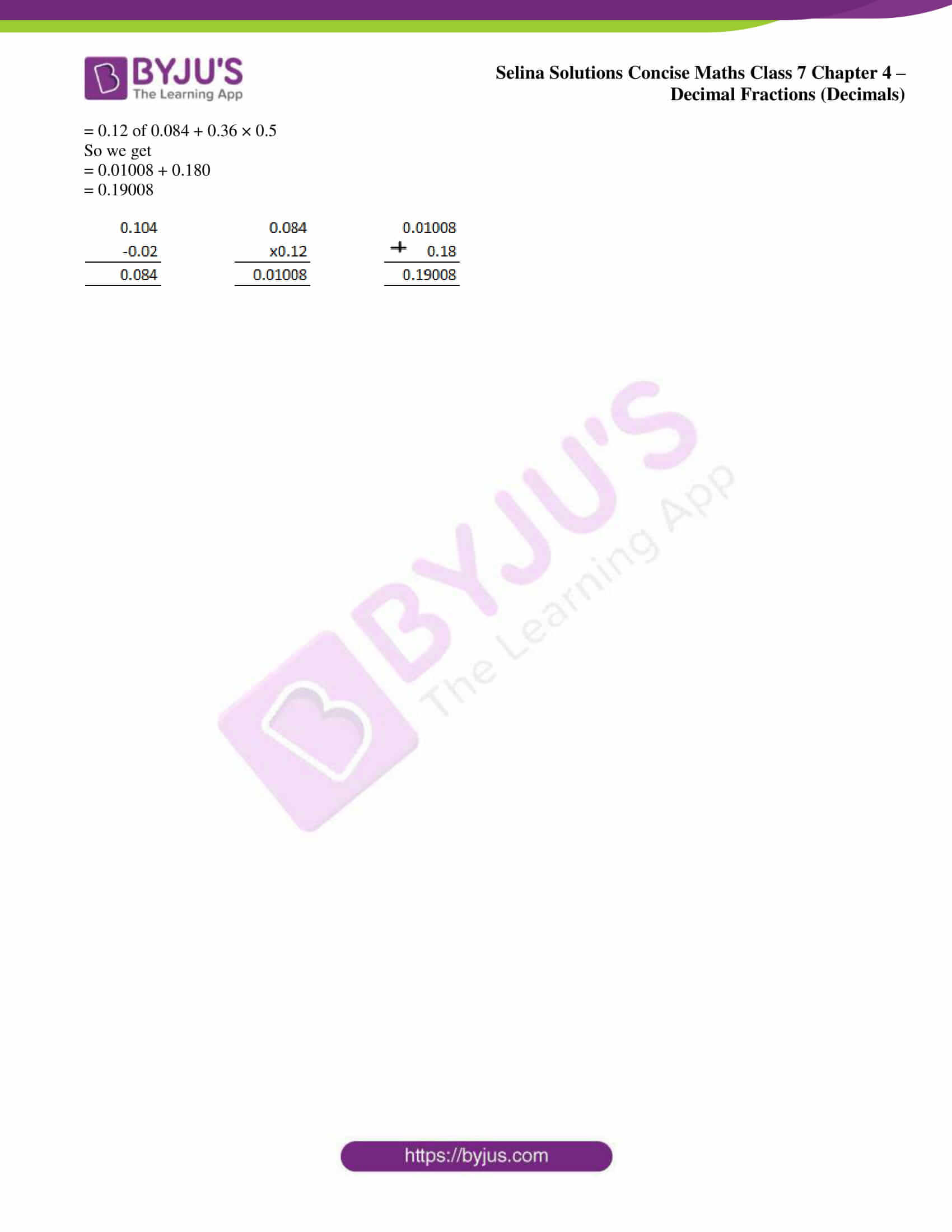### Access Selina Solutions Concise Maths Class 7 Chapter 4: Decimal Fractions (Decimals) Exercise 4F

page: 67

1. The weight of an object is 3.06 kg. Find the total weight of 48 similar objects.

Solution:

It is given that

Weight of an object = 3.06 kg

So the weight of 48 objects = 3.06 × 48 = 146.88 kg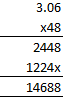2. Find the cost of 17.5 m cloth at the rate of ₹ 112.50 per metre.

Solution:

It is given that

Cost of cloth per metre = ₹ 112.50

So the cost of 17.5 m cloth = ₹ 112.50 × 17.5

On further calculation

= ₹ 1968.750

= ₹ 1968.75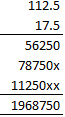3. One kilogramme of oil costs ₹ 73.40. Find the cost of 9.75 kilogramme of the oil.

Solution:

It is given that

Cost of 1 kg oil = ₹ 73.40

So the cost of 9.75 kg oil = ₹ 73.40 × 9.75

On further calculation

= ₹ 715.6500

= ₹ 715.65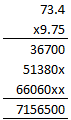4. Total weight of 8 identical objects is 51.2 kg. Find the weight of each object.

Solution:

It is given that

Weight of 8 identical objects = 51.2 kg

So the weight of 1 object = 51.2 ÷ 8 = 6.4 kg

5. 18.5 m of cloth costs ₹ 666. Find the cost of 3.8 m cloth.

Solution:

It is given that

Cost of 18.5 m cloth = ₹ 666

So the cost of 1m cloth = ₹ 666 ÷ 18.5 and cost of 3.8 m cloth

We can write it as

= (666 ÷ 18.5) × 3.8

Multiplying by 10

= (6660 ÷ 185) × 3.8

= 36 × 3.8

So we get

= ₹ 136.80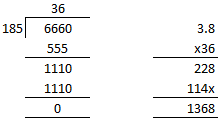6. Find the value of:

(i) 0.5 of ₹ 7.60 + 1.62 of ₹ 30

(ii) 2.3 of 7.3 kg + 0.9 of 0.48 kg

(iii) 6.25 of 8.4 – 4.7 of 3.24

(iv) 0.98 of 235 – 0.09 of 3.2

Solution:

(i) 0.5 of ₹ 7.60 + 1.62 of ₹ 30

It can be written as

= ₹ 3.80 + ₹ 48.60

So we get

= ₹ 52.40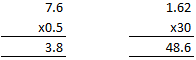(ii) 2.3 of 7.3 kg + 0.9 of 0.48 kg

It can be written as

= 16.79 kg + 0.432 kg

So we get

= 17.222 kg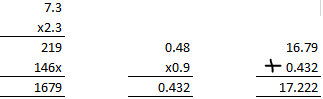(iii) 6.25 of 8.4 – 4.7 of 3.24

It can be written as

= 52.500 – 15.228

So we get

= 37.272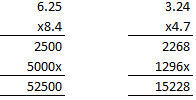(iv) 0.98 of 235 – 0.09 of 3.2

It can be written as

= 230.30 – 0.288

So we get

= 230.012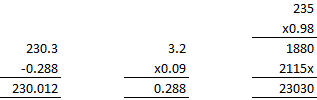7. Evaluate:

(i) 5.6 – 1.5 of 3.4

(ii) 4.8 ÷ 0.04 of 5

(iii) 0.72 of 80 ÷ 0.2

(iv) 0.72 ÷ 80 of 0.2

(v) 6.45 ÷ (3.9 – 1.75)

(vi) 0.12 of (0.104 – 0.02) + 0.36 × 0.5

Solution:

(i) 5.6 – 1.5 of 3.4

It can be written as

= 5.6 – 5.1

So we get

= 0.5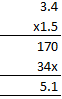(ii) 4.8 ÷ 0.04 of 5

It can be written as

= 4.8 ÷ 0.20

Multiplying by 10

= 48 ÷ 2

= 24

(iii) 0.72 of 80 ÷ 0.2

It can be written as

= 57.60 ÷ 0.2

Multiplying by 10

= 576 ÷ 2

= 288

(iv) 0.72 ÷ 80 of 0.2

It can be written as

= 0.72 ÷ 16.0

Multiplying by 100

= 72 ÷ 1600

= 0.045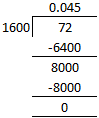(v) 6.45 ÷ (3.9 – 1.75)

It can be written as

= 6.45 ÷ 2.15

Multiplying by 100

= 645 ÷ 215

= 3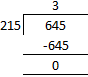(vi) 0.12 of (0.104 – 0.02) + 0.36 × 0.5

It can be written as

= 0.12 of 0.084 + 0.36 × 0.5

So we get

= 0.01008 + 0.180

= 0.19008